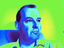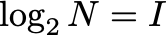top of page
Search
•Richard Harvey

# Information revisited

In my first Gresham Lecture I blithely introduced the concept of Shannon Information and hence Shannon Entropy without much justification . There is a justification tucked away in the transcript but somebody asked me for more so here we go.

Shannon justifies his definition from a set of desiderata (things we want or need to be true). He argues, if these things need to be true then I am going to prove to you, Dear Reader, that this definition of Entropy is the only one possible. Professors like that sort of reasoning but it doesn't help people trying to understand the concept in the first place so let's try another approach.

Let's imagine I asked you how many outcomes a single bit could represent? Well a single bit can be either zero or one hence two outcomes. A two bits? Well you might count them...00, or 01 or 10 or 11. That is the possibilities so four outcomes. And three bits? Well this is becoming a bit tedious but 000, 001, 010, 011, 100, 101, 110, 111 so eight possibilities.

And what if I had I bits? Well by now I expect you have spotted the general pattern which is that if we have I bits then there are N outcomes where:Now at this point we can take logarithms of both sides.I've written a general logarithm, to base a, but if I use a log to base 2 (a = 2) then we can use the fact that log to the base 2 of 2 is 1 soor, if you prefer,But p, the probability of something happening is 1/N so that gives usThe Information, I, is the negative of the log of the probability. The Entropy is nice and simple, it is the mean information. So if we have n events n = 1...N then the mean information iswhich is Shannon's famous equation.

Let's just try a little thought experiment to check it through. Let's imagine we have two outcomes which we label with 0 or 1. And they are equally likely so that N = 2, p1 = 0.5 and p2 = 0.5. So I1 = I2 = - log2(0.5) = 1 so H = 1 bit. Correct!

But what if the two symbols were not equally likely? What is zeros were much more likely? Well in that case the Entropy is less than one which implies that, in principle we could use less than 1 bit to send the information. How can we do that? Well that's another story - the story of compression.

1. A mathematical theory of communication, S E Shannon, Bell System Technical Journal, 27, (3), : 379-423, July 1948.